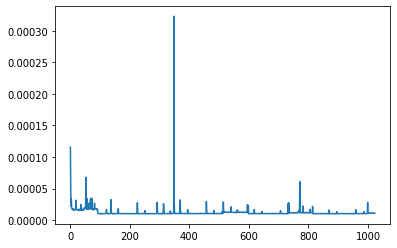# Understanding Variations on Executing Time on GPU

I have the following code snippet.

``````import time
import torch
import numpy as np
import matplotlib.pyplot as plt

from scripts.custom.functional.python.functional_operations import get_functional_operation

device = torch.device('cuda' if torch.cuda.is_available() else 'cpu')
input = torch.randint(32, size=(128, 32, 64, 64), dtype=torch.float, device=device, requires_grad=True)
weights = torch.randint(32, size=(64, 32, 5, 5), dtype=torch.float, device=device, requires_grad=True)

n_rounds = 1024
duration = np.zeros((n_rounds))
conv2d = get_functional_operation('fft-custom')
for i in range(n_rounds):
start = time.time()
output = conv2d(input, weights)
if torch.cuda.is_available(): torch.cuda.synchronize()
duration[i] = time.time() - start
``````

I got assigned the `Tesla V100-SXM2...` and using the Torch Version `1.9.0+cu102`.

I ran this code once and got the following plot for `duration`Then I reset my kernel and ran it again, resulting on the following plot.As part of my research I have to measure the time of the functions I am working with, I am wonder what makes these oscillations on the execution time, even on an extremely controlled and unpractical scenario like the one above were the exact same function with the exact same parameters are evaluated over and over.

Would that be cause because I am using a virtual environment that does not guarantee dedicated resources?

[EDIT]

By the way, how does the graph execution gets optimized anyways? PyTorch is purely on lazy-execution, right? I noticed that usually the first couple of interactions are slower, kind of the execution is still being optimized or it is “warming up”. So, when measuring time I usually disregard the first `128` iterations to be safe. Is there a fixed number of “warming up” iterations?

PyTorch executes eagerly by default. You might be referring to the asynchronous execution of kernel launches on GPUs, where the interpreter will get back to you right away even if the kernel hasn’t finished yet.

There isn’t really a fixed number of warmup iterations, but the reason that they are necessary is that in cases when benchmarking is used to select algorithms (e.g., with `torch.backends.cudnn.benchmark=True`), the first run will be slower due to the benchmarking overhead. I don’t think there will be any graph-level optimizations without jit: TorchScript — PyTorch 1.9.0 documentation